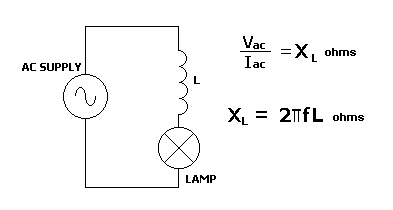Home > Electronic Tutorials > Resistance and Impedance in AC Circuit > Inductive Reactance Tutorial

Resistance and Impedance in AC Circuit

# Inductive Reactance TutorialThe coil opposes the flow of ac current, as a resistor does in a dc circuit.  This opposition is called inductive reactance, XL. It is measured in ohms.  Ohm's Law can be applied, as in the top formula.

The bottom formula shows how inductive reactance is calculated.

f is the frequency of the applied voltage, and L is the value of the coil in Henries.

It can be seen from this formula that the value of XL goes up as the frequency increases. It also goes up if the value of the coil increases.

This means that as the value of L or f increases, the opposition to the flow of ac current increases, and the lamp will glow less.